# Test: Congruence Criteria- SAS And ASA

## 15 Questions MCQ Test Mathematics (Maths) Class 9 | Test: Congruence Criteria- SAS And ASA

Description
This mock test of Test: Congruence Criteria- SAS And ASA for Class 9 helps you for every Class 9 entrance exam. This contains 15 Multiple Choice Questions for Class 9 Test: Congruence Criteria- SAS And ASA (mcq) to study with solutions a complete question bank. The solved questions answers in this Test: Congruence Criteria- SAS And ASA quiz give you a good mix of easy questions and tough questions. Class 9 students definitely take this Test: Congruence Criteria- SAS And ASA exercise for a better result in the exam. You can find other Test: Congruence Criteria- SAS And ASA extra questions, long questions & short questions for Class 9 on EduRev as well by searching above.
QUESTION: 1

### In the given figure, AB = AC, AD = AE = 5 cm and DC = 8 cm. Length of EB is.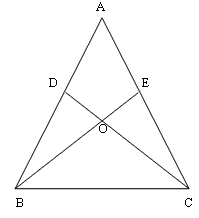Solution:

8 cm is the answer because DC =EB=8 cm.

QUESTION: 2

Solution:
QUESTION: 3

### Which of the following statements is incorrect ?

Solution:

2 rectangles having same area may differ in their lengths. So they will not be congruent

QUESTION: 4

In the following figure, PQ = PR and SQ = SR, then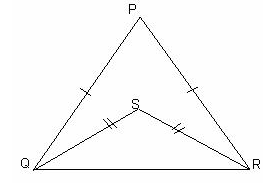Solution:
QUESTION: 5

If two triangles ABC and PQR are congruent under the correspondence A ↔ P, B ↔ Q and C ↔ R, then symbolically, it is expressed as

Solution:
QUESTION: 6

If ΔABC ≌ ΔPQR then which of the following is true:

Solution:

Corresponding sides in congruent triangles are equal .

So AC=PR ,AB=PQ ,BC=QR

QUESTION: 7

If two sides of a triangle are equal, the angles opposite to these sides are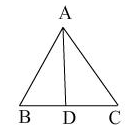Solution:

Theorem: Angles opposite to equal sides of an isosceles triangle are equal.

QUESTION: 8

In case of two equilateral triangles, PQR and STU which of the following correspondence is not correct?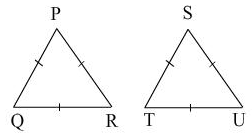Solution:

The correct option is Option A.

All equilateral triangles have the same angles by the congruence rule (SSS)

So, TTS <—> PQR

QUESTION: 9

In quadrilateral ADBC, AB bisects ∠A. Which of the following criterion will prove ΔABC ≅ ΔABD?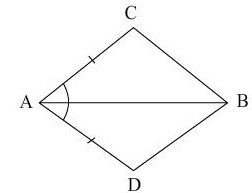Solution:

and AB being the bisector of ∠A.

Now, in ΔABC and ΔABD,
[Given]
AB = AB
[Common]
∠CAB = ∠DAB [∴ AB bisects ∠CAD]

∴ Using SAS criteria, we have
ΔABC ≌ ΔABD.

∴ Corresponding parts of congruent triangles (c.p.c.t) are equal.
∴ BC = BD

QUESTION: 10

In an isosceles triangle ABC with AB = AC, if BD and CE are the altitudes, then BD and CE are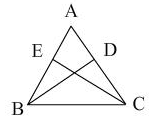Solution:
QUESTION: 11

In fig., if AB = AC and PB = QC, then by which congruence criterion PBC ≅ QCB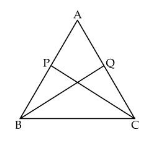Solution:

As AB = AC so angle ACB = angle(ABC) as angles opposite to equal sides r equal.

In triangle PBC and Triangle QCB we see that

I) PB = QC (given)

ii) angle(PBC) = (angle)QCB (proved earliar)

iii) BC = BC (common)

so triangle PBC is congruent to triangle QCB by SAS axiom of congruency.

QUESTION: 12

The diagonal PR of a quadrilateral PQRS bisects the angles P and R, then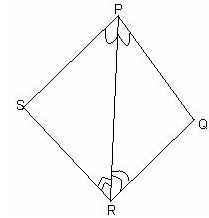Solution:

In Δ PSR and ΔPQR

PR = PR

∠ 1 = ∠ 2

∠ 3 = ∠ 4

Δ PSR ≅ Δ PQR  [ASA]

PS = PQ   [CPCT]

QR = RS  [CPCT]

The correct option is Option A

QUESTION: 13

In the given figure, AB = EF, BC = DE, AB ⊥ BD and EF ⊥ CE. Which of the following criterion is true for ΔABD ≅ ΔEFC?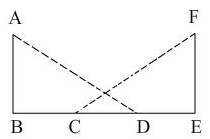Solution:
QUESTION: 14

Two figures are congruent if they have

Solution:

In geometry, two figures or objects are congruent if they have the same shape and size, or if one has the same shape and size as the mirror image of the other.

QUESTION: 15

A triangle can have:

Solution:

The least number of acute angles that a triangle can have is 2.

As we cannot have more than one right angle or obtuse angle, we have only two or three acute angles in a triangle.

Further, if one angle is acute, sum of other two angles is more than 90 deg and we cannot have two right angles or obtuse angles.